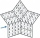# Reason + natural numbers - math problems

#### Number of problems found: 211

• DigitsHow many natural numbers greater than 4000 which are formed from the numbers 0,1,3,7,9 with the figures not repeated, B) How many will the number of natural numbers less than 4000 and the numbers can be repeated?
• Unknown numbersThe sum of two consecutive natural numbers and their triple is 92. Find these numbers.
• Big numbersHow many natural numbers less than 10 to the sixth can be written in numbers: a) 9.8.7 b) 9.8.0
• All pairsDetermine all pairs (m, n) of natural numbers for which is true: m s (n) = n s (m) = 70, where s (a) denotes the digit sum of the natural number a.
• AM of three numbersThe number 2010 can be written as the sum of 3 consecutive natural numbers. Determine the arithmetic mean of these numbers.
• Find twoFind two consecutive natural numbers whose product is 1 larger than their sum. Searched numbers expressed by a fraction whose numerator is the difference between these numbers and the denominator is their sum.
• Three-digitHow many do three-digit natural numbers not have the number 7?
• Divisible by nineHow many three-digit natural numbers in total are divisible without a remainder by the number 9?
• Number 4Kamila wrote all natural numbers from 1 to 400 inclusive. How many times did she write the number 4?
• Twenty-fiveHow many are three-digit natural numbers divisible by 25?
• Sum of the digitsHow many are two-digit natural numbers that have the sum of the digits 9?
• Sum of the seventeen numbersThe sum of the 17 different natural numbers is 154. Determine the sum of the two largest ones.
• Find the sumFind the sum of all natural numbers from 1 and 100, which are divisible by 2 or 5
• Z9–I–1In all nine fields of given shape to be filled natural numbers so that: • each of the numbers 2, 4, 6, and 8 is used at least once, • four of the inner square boxes containing the products of the numbers of adjacent cells of the outer square, • in the cir
• Reminder and quotientThere are given the number C = 281, D = 201. Find the highest natural number S so that the C:S, D:S are with the remainder of 1,
• Three-digit numbersUse the numbers 4,5,8,9 to write all three-digit numbers without repetition. How many such numbers are there?
• RectanglesThe perimeter of a rectangle is 90 m. Divide it into three rectangles, the shorter side has all three rectangles the same, their longer sides are three consecutive natural numbers. What is the dimensions of each rectangle?
• Odd numbersThe sum of four consecutive odd numbers is 1048. Find those numbers ...
• Three-digit numbersWe have digits 0,1,4,7 that cannot be repeated. How many three-digit numbers can we write from them? You can help by listing all the numbers.
• How manyHow many numbers are less than 222 with a digit sum is 8?

Do you have an interesting mathematical word problem that you can't solve it? Submit a math problem, and we can try to solve it.

We will send a solution to your e-mail address. Solved examples are also published here. Please enter the e-mail correctly and check whether you don't have a full mailbox.

Please do not submit problems from current active competitions such as Mathematical Olympiad, correspondence seminars etc...
Reason - math problems. Natural numbers - math problems.